Ch.1 - Intro to General Chemistry 2hrs & 53mins 0% complete Worksheet Ch.2 - Atoms & Elements 2hrs & 49mins 0% complete Worksheet Ch.3 - Chemical Reactions 3hrs & 25mins 0% complete Worksheet BONUS: Lab Techniques and Procedures 1hr & 38mins 0% complete Worksheet BONUS: Mathematical Operations and Functions 47mins 0% complete Worksheet Ch.4 - Chemical Quantities & Aqueous Reactions 3hrs & 30mins 0% complete Worksheet Ch.5 - Gases 3hrs & 47mins 0% complete Worksheet Ch.6 - Thermochemistry 2hrs & 28mins 0% complete Worksheet Ch.7 - Quantum Mechanics 2hrs & 35mins 0% complete Worksheet Ch.8 - Periodic Properties of the Elements 1hr & 57mins 0% complete Worksheet Ch.9 - Bonding & Molecular Structure 2hrs & 5mins 0% complete Worksheet Ch.10 - Molecular Shapes & Valence Bond Theory 1hr & 31mins 0% complete Worksheet Ch.11 - Liquids, Solids & Intermolecular Forces 3hrs & 40mins 0% complete Worksheet Ch.12 - Solutions 2hrs & 17mins 0% complete Worksheet Ch.13 - Chemical Kinetics 2hrs & 22mins 0% complete Worksheet Ch.14 - Chemical Equilibrium 2hrs & 26mins 0% complete Worksheet Ch.15 - Acid and Base Equilibrium 4hrs & 42mins 0% complete Worksheet Ch.16 - Aqueous Equilibrium 3hrs & 48mins 0% complete Worksheet Ch. 17 - Chemical Thermodynamics 1hr & 44mins 0% complete Worksheet Ch.18 - Electrochemistry 2hrs & 58mins 0% complete Worksheet Ch.19 - Nuclear Chemistry 1hr & 33mins 0% complete Worksheet Ch.20 - Organic Chemistry 3hrs 0% complete Worksheet Ch.22 - Chemistry of the Nonmetals 2hrs & 1min 0% complete Worksheet Ch.23 - Transition Metals and Coordination Compounds 1hr & 54mins 0% complete Worksheet

# Ideal Gas Law

See all sectionsJules Bruno

The Ideal Gas Law represents a formula that relates the behavior of gases under varying conditions of concentration, volume, pressure and temperature.

The Ideal Gas Law

Within a closed container, gas molecules behave ideally when the temperature and volume are high while the pressure is low. The Ideal Gas Law equation is represented as: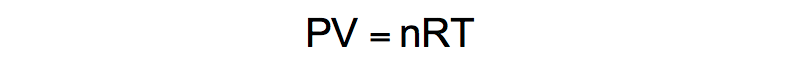Ideal Gas Law

R Constant (R)

The variable of R represents the universal gas constant of the gas. When atmospheres (atm) are incorporated into its units the traditional R value of 0.08206 is used, but changing atmospheres (atm) will change the numerical value of R.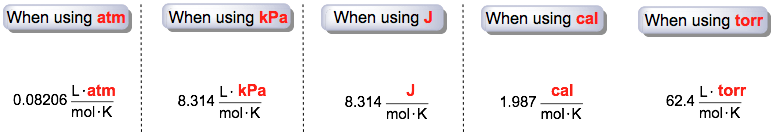Universal R Constant (Different Forms)

Pressure (P)

The variable of P represents the pressure of the gas. Although the SI unit for pressure is pascals, it is customary to use the units of atmospheres (atm).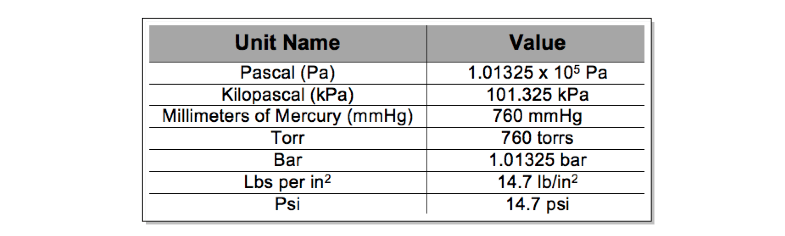Units of Pressure

1.0 atmosphere (atm) is equal to each of the given pressure units based on the given value. For example, 1.0 atm = 760 torrs or 1.0 atm = 101.325 kPa.

Volume (V)

The variable of V represents the volume of the gas. The units are in Liters.

Temperature (T)

The variable of T represents the absolute temperature of the gas. The units are in Kelvin. Let’s say you are given 90oF and asked to convert it into Kelvin.

Conversion from Fahrenheit to Celsius: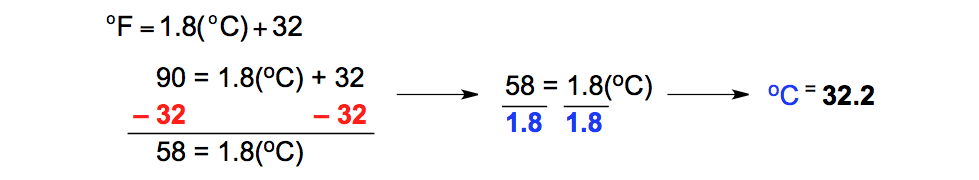Fahrenheit to Celsius ConversionConversion from Celsius to Kelvin:Celsius to Kelvin Conversion

Moles (n)

The variable of n represents the moles of the gas.

PRACTICE:

How many grams of neon gas are present in a 5.0 L container at pressure of 1.35 atm at 35oC?

STEP 1: Identify the variables given.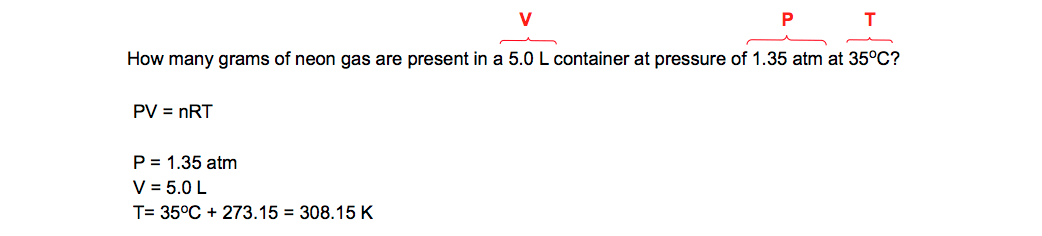Problem Outline

STEP 2: Isolate the missing variable of moles (n).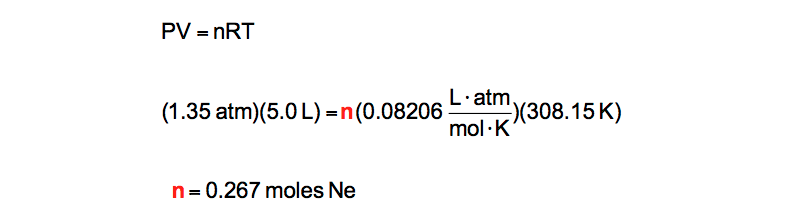Solving for moles (n)

STEP 3: Convert the moles (n) into grams.Moles to Grams Conversion

Further Applications of the Ideal Gas Law

The Ideal Gas Law can be rearranged in order to create new equations that determine either the density or molar mass of a gas molecule.

When dealing with the density of a gas molecule we can utilize the following equation:Derived Gas Law (Density)

Pressure, R constant and Temperature

The variables of P, R and T still represent pressure, the gas constant, and temperature in Kelvin.

Molar Mass (M)

The variable of M represents the molar mass of a gas molecule. The units of molar mass are grams per mole (g/mol).

Density (d)

The variable of d represents the density of a gas molecule. As a result of being less dense than solids or liquids, gas molecules have their density in units of grams per liter (g/L).

PRACTICE: Determine the density of NH3 gas at 435 K and 1.00 atm.

STEP 1: Identify the variables given.Identify given variables

STEP 2: Solve for the missing variable of density (d).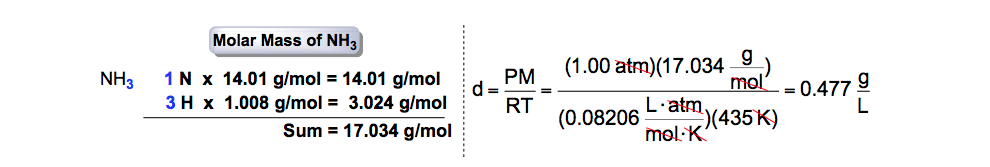Solving for missing variable (density)

When dealing with the mass and molar mass of a gas molecule we can utilize the following equation:Derived Gas Law (Molar Mass)

Pressure, Volume, R constant, Molar Mass and Temperature

The variables of P,V, M, R and T are the same ones used from the previous equations.

Mass (m)

The variable of m represents the mass of a gas molecule. The units of mass are grams (g).

PRACTICE: An unknown gas contained in a 1.85 L flask is weighed and found to have a mass of 15.87 g at a pressure of 2.45 atm and a temperature of 18.6°C. What is the molecular weight of the gas?

STEP 1: Identify the variables given.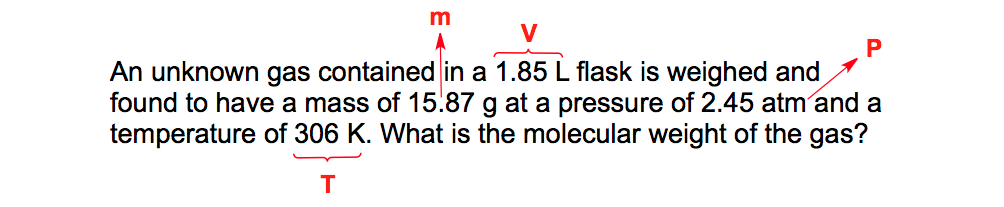Identify given variables

STEP 2: Isolate the missing variable of molar mass (M).Solving for missing variable (molar mass)Beyond Ideal Gases

To better understand the reactive nature of gases you can utilize the Kinetic-Molecular Theory and the Van der Waals equation. They help to explain concepts such as effusion rates of gasesDalton’s LawRoot Mean Square Speed, and the Simple Gas LawsJules Bruno

Jules felt a void in his life after his English degree from Duke, so he started tutoring in 2007 and got a B.S. in Chemistry from FIU. He’s exceptionally skilled at making concepts dead simple and helping students in covalent bonds of knowledge.